Anzeige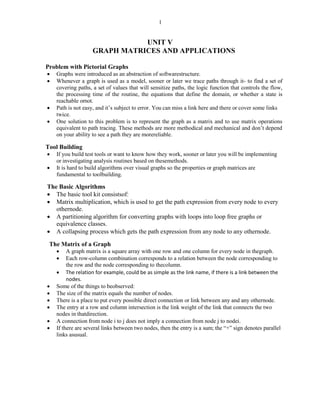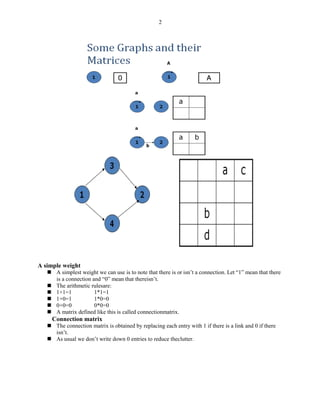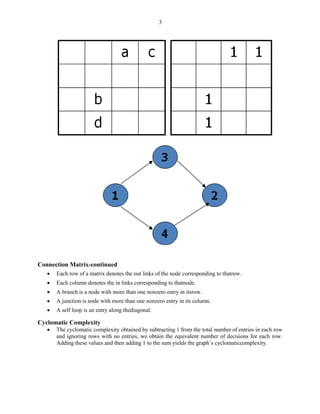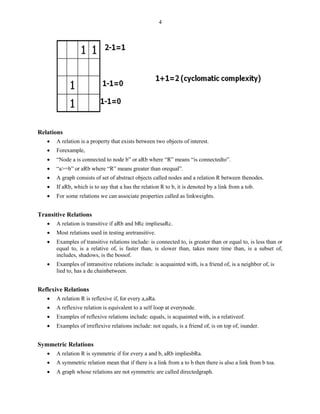Anzeige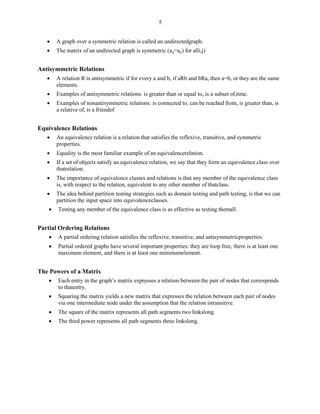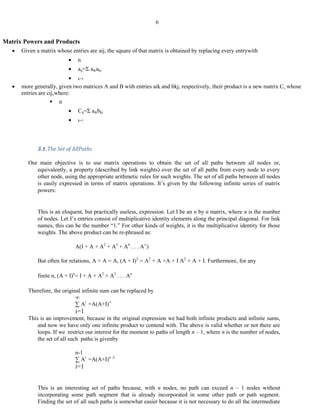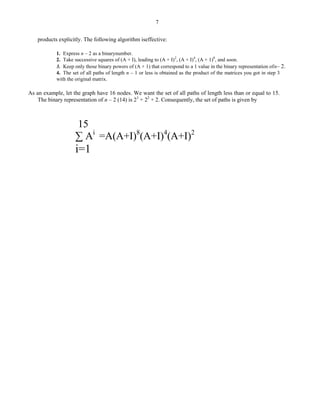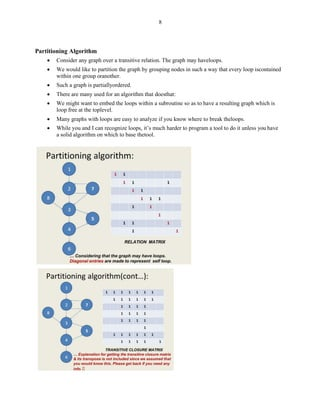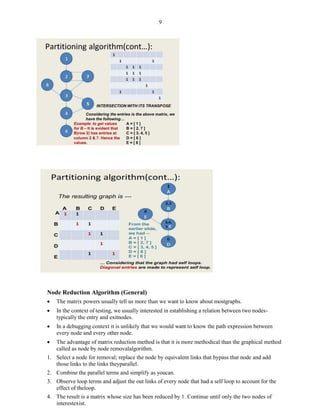Anzeige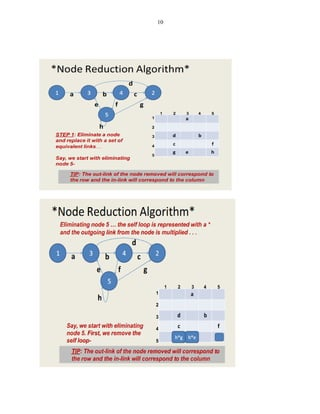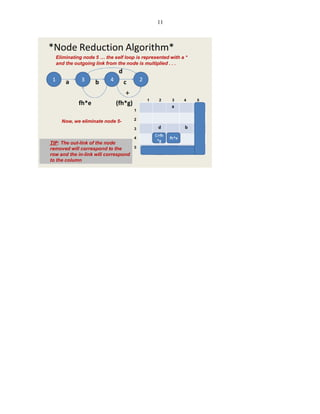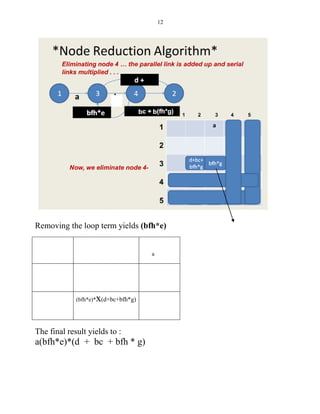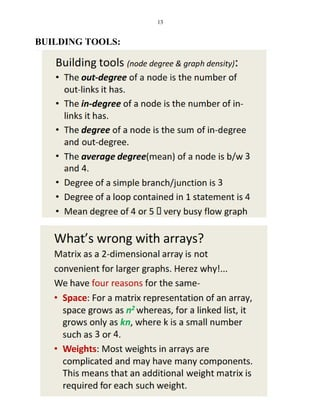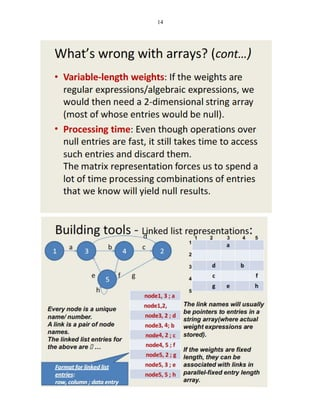Anzeige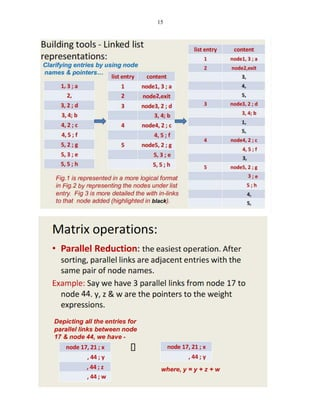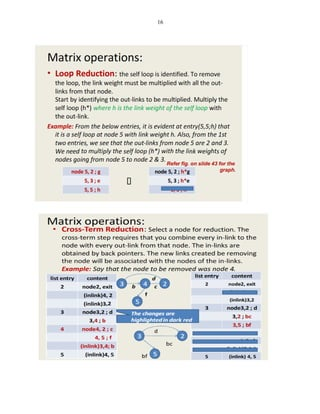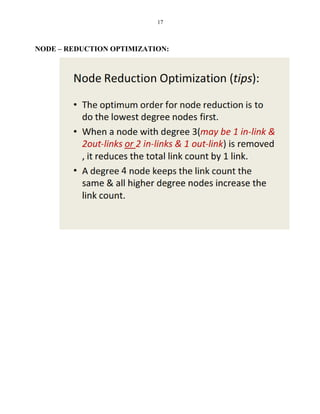Nächste SlideShare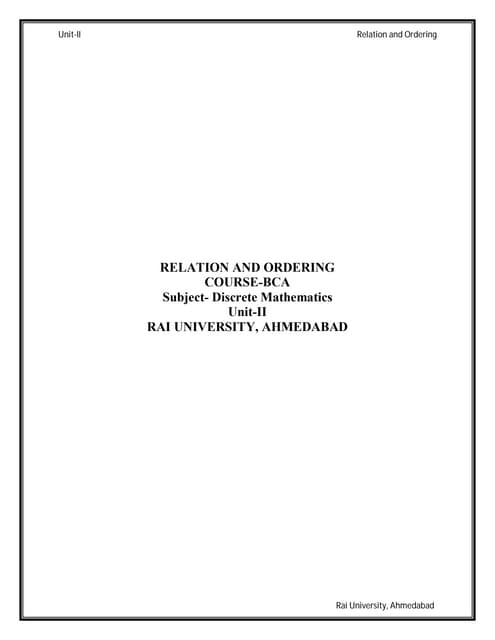BCA_Semester-II-Discrete Mathematics_unit-ii_Relation and ordering
1 von 17
Anzeige

### GRAPH MATRIX APPLICATIONS.pdf

1. 1 UNIT V GRAPH MATRICES AND APPLICATIONS Problem with Pictorial Graphs  Graphs were introduced as an abstraction of softwarestructure.  Whenever a graph is used as a model, sooner or later we trace paths through it- to find a set of covering paths, a set of values that will sensitize paths, the logic function that controls the flow, the processing time of the routine, the equations that define the domain, or whether a state is reachable ornot.  Path is not easy, and it’s subject to error. You can miss a link here and there or cover some links twice.  One solution to this problem is to represent the graph as a matrix and to use matrix operations equivalent to path tracing. These methods are more methodical and mechanical and don’t depend on your ability to see a path they are morereliable. Tool Building  If you build test tools or want to know how they work, sooner or later you will be implementing or investigating analysis routines based on thesemethods.  It is hard to build algorithms over visual graphs so the properties or graph matrices are fundamental to toolbuilding. The Basic Algorithms  The basic tool kit consistsof:  Matrix multiplication, which is used to get the path expression from every node to every othernode.  A partitioning algorithm for converting graphs with loops into loop free graphs or equivalence classes.  A collapsing process which gets the path expression from any node to any othernode. The Matrix of a Graph  A graph matrix is a square array with one row and one column for every node in thegraph.  Each row-column combination corresponds to a relation between the node corresponding to the row and the node corresponding to thecolumn.  The relation for example, could be as simple as the link name, if there is a link between the nodes.  Some of the things to beobserved:  The size of the matrix equals the number of nodes.  There is a place to put every possible direct connection or link between any and any othernode.  The entry at a row and column intersection is the link weight of the link that connects the two nodes in thatdirection.  A connection from node i to j does not imply a connection from node j to nodei.  If there are several links between two nodes, then the entry is a sum; the “+” sign denotes parallel links asusual.
2. 2 A simple weight  A simplest weight we can use is to note that there is or isn’t a connection. Let “1” mean that there is a connection and “0” mean that thereisn’t.  The arithmetic rulesare:  1+1=1 1*1=1  1+0=1 1*0=0  0+0=0 0*0=0  A matrix defined like this is called connectionmatrix. Connection matrix  The connection matrix is obtained by replacing each entry with 1 if there is a link and 0 if there isn’t.  As usual we don’t write down 0 entries to reduce theclutter.
3. 3 Connection Matrix-continued  Each row of a matrix denotes the out links of the node corresponding to thatrow.  Each column denotes the in links corresponding to thatnode.  A branch is a node with more than one nonzero entry in itsrow.  A junction is node with more than one nonzero entry in its column.  A self loop is an entry along thediagonal. Cyclomatic Complexity  The cyclomatic complexity obtained by subtracting 1 from the total number of entries in each row and ignoring rows with no entries, we obtain the equivalent number of decisions for each row. Adding these values and then adding 1 to the sum yields the graph’s cyclomaticcomplexity.
4. 4 Relations  A relation is a property that exists between two objects of interest.  Forexample,  “Node a is connected to node b” or aRb where “R” means “is connectedto”.  “a>=b” or aRb where “R” means greater than orequal”.  A graph consists of set of abstract objects called nodes and a relation R between thenodes.  If aRb, which is to say that a has the relation R to b, it is denoted by a link from a tob.  For some relations we can associate properties called as linkweights. Transitive Relations  A relation is transitive if aRb and bRc impliesaRc.  Most relations used in testing aretransitive.  Examples of transitive relations include: is connected to, is greater than or equal to, is less than or equal to, is a relative of, is faster than, is slower than, takes more time than, is a subset of, includes, shadows, is the bossof.  Examples of intransitive relations include: is acquainted with, is a friend of, is a neighbor of, is lied to, has a du chainbetween. Reflexive Relations  A relation R is reflexive if, for every a,aRa.  A reflexive relation is equivalent to a self loop at everynode.  Examples of reflexive relations include: equals, is acquainted with, is a relativeof.  Examples of irreflexive relations include: not equals, is a friend of, is on top of, isunder. Symmetric Relations  A relation R is symmetric if for every a and b, aRb impliesbRa.  A symmetric relation mean that if there is a link from a to b then there is also a link from b toa.  A graph whose relations are not symmetric are called directedgraph.
5. 5  A graph over a symmetric relation is called an undirectedgraph.  The matrix of an undirected graph is symmetric (aij=aji) for alli,j) Antisymmetric Relations  A relation R is antisymmetric if for every a and b, if aRb and bRa, then a=b, or they are the same elements.  Examples of antisymmetric relations: is greater than or equal to, is a subset of,time.  Examples of nonantisymmetric relations: is connected to, can be reached from, is greater than, is a relative of, is a friendof Equivalence Relations  An equivalence relation is a relation that satisfies the reflexive, transitive, and symmetric properties.  Equality is the most familiar example of an equivalencerelation.  If a set of objects satisfy an equivalence relation, we say that they form an equivalence class over thatrelation.  The importance of equivalence classes and relations is that any member of the equivalence class is, with respect to the relation, equivalent to any other member of thatclass.  The idea behind partition testing strategies such as domain testing and path testing, is that we can partition the input space into equivalenceclasses.  Testing any member of the equivalence class is as effective as testing themall. Partial Ordering Relations  A partial ordering relation satisfies the reflexive, transitive, and antisymmetricproperties.  Partial ordered graphs have several important properties: they are loop free, there is at least one maximum element, and there is at least one minimumelement. The Powers of a Matrix  Each entry in the graph’s matrix expresses a relation between the pair of nodes that corresponds to thatentry.  Squaring the matrix yields a new matrix that expresses the relation between each pair of nodes via one intermediate node under the assumption that the relation istransitive.  The square of the matrix represents all path segments two linkslong.  The third power represents all path segments three linkslong.
6. 6 Matrix Powers and Products  Given a matrix whose entries are aij, the square of that matrix is obtained by replacing every entrywith  n  aij=Σ aikakj  k=1  more generally, given two matrices A and B with entries aik and bkj, respectively, their product is a new matrix C, whose entries are cij,where:  n  Cij=Σ aikbkj  k=1 3.1.The Set of AllPaths Our main objective is to use matrix operations to obtain the set of all paths between all nodes or, equivalently, a property (described by link weights) over the set of all paths from every node to every other node, using the appropriate arithmetic rules for such weights. The set of all paths between all nodes is easily expressed in terms of matrix operations. It’s given by the following infinite series of matrix powers: This is an eloquent, but practically useless, expression. Let I be an n by n matrix, where n is the number of nodes. Let I’s entries consist of multiplicative identity elements along the principal diagonal. For link names, this can be the number “1.” For other kinds of weights, it is the multiplicative identity for those weights. The above product can be re-phrased as: A(I + A + A2 + A3 + A4 . . . A∞ ) But often for relations, A + A = A, (A + I)2 = A2 + A +A + I A2 + A + I. Furthermore, for any finite n, (A + I)n = I + A + A2 + A3 . . . An. Therefore, the original infinite sum can be replaced by ∞ ∑ Ai =A(A+I)∞ i=1 This is an improvement, because in the original expression we had both infinite products and infinite sums, and now we have only one infinite product to contend with. The above is valid whether or not there are loops. If we restrict our interest for the moment to paths of length n – 1, where n is the number of nodes, the set of all such paths is givenby n-1 ∑ Ai =A(A+I)n -2 i=1 This is an interesting set of paths because, with n nodes, no path can exceed n – 1 nodes without incorporating some path segment that is already incorporated in some other path or path segment. Finding the set of all such paths is somewhat easier because it is not necessary to do all the intermediate
7. 7 products explicitly. The following algorithm iseffective: 1. Express n – 2 as a binarynumber. 2. Take successive squares of (A + I), leading to (A + I)2 , (A + I)4 , (A + 1)8 , and soon. 3. Keep only those binary powers of (A + 1) that correspond to a 1 value in the binary representation ofn– 2. 4. The set of all paths of length n – 1 or less is obtained as the product of the matrices you got in step 3 with the original matrix. As an example, let the graph have 16 nodes. We want the set of all paths of length less than or equal to 15. The binary representation of n – 2 (14) is 23 + 22 + 2. Consequently, the set of paths is given by 15 ∑ Ai =A(A+I)8 (A+I)4 (A+I)2 i=1
8. 8 Partitioning Algorithm  Consider any graph over a transitive relation. The graph may haveloops.  We would like to partition the graph by grouping nodes in such a way that every loop iscontained within one group oranother.  Such a graph is partiallyordered.  There are many used for an algorithm that doesthat:  We might want to embed the loops within a subroutine so as to have a resulting graph which is loop free at the toplevel.  Many graphs with loops are easy to analyze if you know where to break theloops.  While you and I can recognize loops, it’s much harder to program a tool to do it unless you have a solid algorithm on which to base thetool.
9. 9 Node Reduction Algorithm (General)  The matrix powers usually tell us more than we want to know about mostgraphs.  In the context of testing, we usually interested in establishing a relation between two nodes- typically the entry and exitnodes.  In a debugging context it is unlikely that we would want to know the path expression between every node and every other node.  The advantage of matrix reduction method is that it is more methodical than the graphical method called as node by node removalalgorithm. 1. Select a node for removal; replace the node by equivalent links that bypass that node and add those links to the links theyparallel. 2. Combine the parallel terms and simplify as youcan. 3. Observe loop terms and adjust the out links of every node that had a self loop to account for the effect of theloop. 4. The result is a matrix whose size has been reduced by 1. Continue until only the two nodes of interestexist.
10. 10
11. 11
12. 12 Removing the loop term yields (bfh*e) a (bfh*e)*x(d+bc+bfh*g) The final result yields to : a(bfh*e)*(d + bc + bfh * g)
13. 13 BUILDING TOOLS:
14. 14
15. 15
16. 16
17. 17 NODE – REDUCTION OPTIMIZATION:
Anzeige